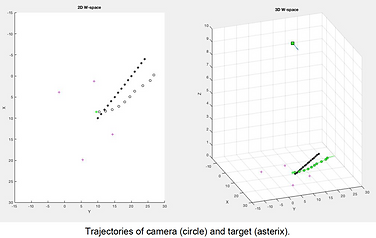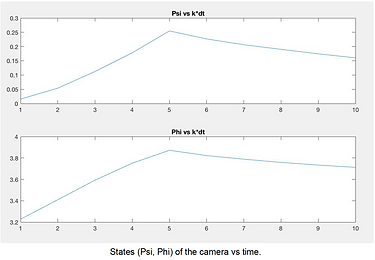## Skills

• Matlab

• Sensor Planning

• Optimal Control

• State Estimation

## Fall, 2017###### 01 description

This project addresses the problem of tracking a moving object in a 2D workspace W  using a pan & tilt camera.  The camera is located in the center of a room at a height h and the object has unknown dynamics.   The state of the camera is represented with a vector s and a control vector u that changes the shape of the FOV in by moving the camera. ​

Given the assumptions above the algorithm calculates the control vector u at each time step to optimize the visibility of the target.   Control algorithm and simulations were implemented in Matlab.### Representation of the workspace used in the project.  Provided by Prof. Silvia Ferrari.

###### 3 implementation

An optimal control method can be used to determine the control input u of the camera for a moving target by minimizing a cost function that penalizes the distance from the center of the FOV and the predicted position of the target.  Using the pinhole camera model, it is possible to represent any point in the 3D space into the camera fixed frame.### Examples of results for tested trajectory for a target moving in a straight trajectory at variable acceleration (left), and a target moving at variable speed while rapidly changing heading direction (right).

###### 4 acknowledgement

This work was developed under the supervision of Prof. Silvia Ferrari as part of MAE 6790: Intelligent Sensor Planning.